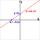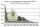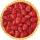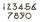# Examples of equations for 7th grade (12y)

#### Number of problems found: 560

• Simple equation 324 = n • 27, solve for n
• Wood dividingWhich equation calculates the number of 1/3-foot pieces that can be cut from a piece of wood that is 7 feet long?
• RootThe root of the equation ? is (equal or greater or less than zero)? ?
• FruitsAmy bought a basket of fruits 1/5 of them were apples,1/4 were oranges, and the rest were 33 bananas. How many fruits did she buy in all?
• Fraction + eqSolve following simple equation with fractions: -5/6(8+5b) = 75 + 5/3b
• TaxiA taxi ride costs \$3 plus \$2.50 per mile. Write and Graph an equation in two variables that represents the total cost of a taxi ride.
• One thirdIf 3/5 is 360, how much is 1/3?
• GivenGiven 2x =0.125 find the value of x
• Christmas or EasterPlease calculate this example by the Venn equation. They asked 73 students whether they like Christmas or Easter. 34 of them like one of the holidays. 39 loves Easter. There are twice as many students who wish both holidays than those who only love Easter
• InflationWhat is better for people (employees)? ?
• WhichWhich decimals when subtracted equal 3.89: a - b = 3.89
• TimeageSeven times of my age is 8 less than the largest two-digit number. How old I am?
• Unknown numberI think the number - its sixth is 3 smaller than its third.
• Strange xFor what x is true ??
• Unknown number 6Determine x if 1/6 of x is equal to 2/5 of the number 24.
• The quotientThe quotient of g and 55 is the same as 279. What is g?
• Midpoint 6FM=8a+1, FG=42, a=? Point M is the midpoint of FG. Find unknown a.
• NumberWhat number I think? The third is 6 and half is 2 more than the its quarter.
• ExpressionIf it is true that ? is:
• BoatsThree-quarters of boats are white, 1/7 are blue and 9 are red. How many boats do we have?

Do you have an interesting mathematical word problem that you can't solve it? Submit a math problem, and we can try to solve it.

We will send a solution to your e-mail address. Solved examples are also published here. Please enter the e-mail correctly and check whether you don't have a full mailbox.

Please do not submit problems from current active competitions such as Mathematical Olympiad, correspondence seminars etc...

Do you have a linear equation or system of equations and looking for its solution? Or do you have quadratic equation? Equations Math problems. Examples for 7th grade (seventh).